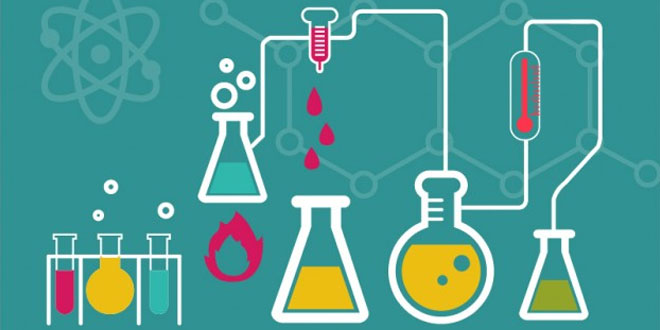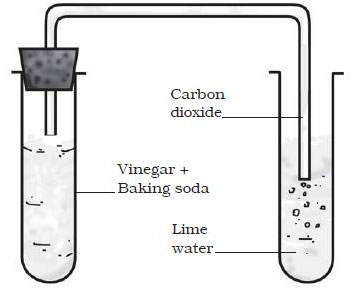Thursday , July 7 2022# NCERT 7th Class (CBSE) Science: Chemical and Chemical Changes

#### Question: Write the steps to balance a chemical equation. Explain it with example.

Answer: Let us consider the chemical reaction of magnesium with oxygen.  Mg + O2 → MgO. The steps to balance this Chemical equation is as follows:

Count the number of atoms of each element in the reactants and products of the chemical equation.

Element
Number of atoms in reactants (LHS)
Number of atoms in products (RHS)
Mg
1
1
O
2
1

In this equation we see that one atom of magnesium and two atoms of oxygen take part in the reaction to form one molecule of Magnesium oxide. But one oxygen atom is enough to react with magnesium to form one molecule of magnesium oxide. Oxygen cannot be destroyed therefore two atoms of oxygen are participating in the reaction. Each will need a magnesium atom. So we balance the equation to show the correct number of atoms participating in the reaction.

To balance oxygen atoms on both sides of the equation, multiply the product (RHS) by 2.

Element
Number of atoms in reactants (LHS)
Number of atoms in products (RHS)
Mg
1
1×2=2
O
2
1×2=2
Mg (Magnesium) + O2 (Oxygen)→ 2MgO (Magnesium oxide)

Now, in the equation, the number of oxygen atoms is balanced but the number of magnesium atoms on both sides is not balanced.

To balance magnesium atoms on both sides of the equation, multiply the magnesium atom in the reactants (LHS) by 2.

Element
Number of atoms in reactants (LHS)
Number of atoms in products (RHS)
Mg
1×2=2
1×2=2
O
2
1×2=2
2Mg (Magnesium) + O2 (Oxygen)→ 2MgO (Magnesium oxide)

Now the above chemical equation is said to be balanced.

#### Question: What happens when Vinegar and baking soda is mixed.

Answer: Reaction between Vinegar and Baking soda:

1. Take vinegar (acetic acid) in a glass beaker and add a pinch of baking soda (Sodium hydrogen carbonate) to it.
2. You will see bubbles coming out of beaker.
3. This is an example of chemical change as the formation of gas bubbles is due to release of carbon dioxide.
4. This can be confirmed by making the gas produced pass through freshly prepared lime water.
5. The lime water turn milky when carbon dioxide is passed through it due to formation of Calcium Carbonate.

Vinegar + Baking soda → Carbon dioxide + water + other compoundsThe chemical reaction can be represented by the following equation:

Carbon dioxide (CO2 + Lime water [Ca(OH)2 → Calcium carbonate (CaCO33) + Water (H2O)

## Sources of Energy: 10th Science Chapter 14

Class: 10th Class Subject: Science Chapter: Chapter 14: Sources of Energy Quiz: – Questions MCQs: …

### One comment

1.I like this site. It is very good.